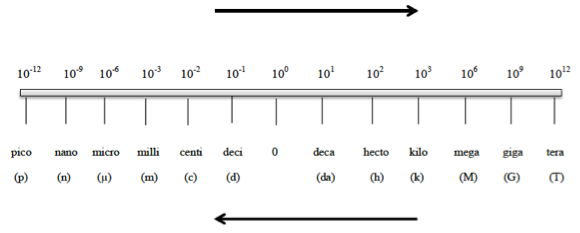# Problem: Use the prefix multipliers to express each of the following measurements without exponents.1.5 x 103 g

###### FREE Expert Solution

Metric Prefixes are “labels” that can be placed in front of base units. Whenever using the metric prefix chart, remember that “1” is associated with the metric prefixConversion factor needed:

1 kg = 103 g

91% (436 ratings)###### Problem Details

Use the prefix multipliers to express each of the following measurements without exponents.

1.5 x 103 g

Frequently Asked Questions

What scientific concept do you need to know in order to solve this problem?

Our tutors have indicated that to solve this problem you will need to apply the Metric Prefixes concept. You can view video lessons to learn Metric Prefixes. Or if you need more Metric Prefixes practice, you can also practice Metric Prefixes practice problems.